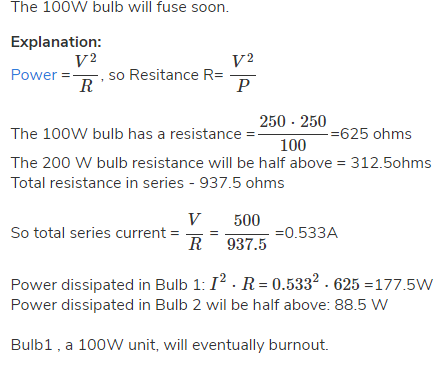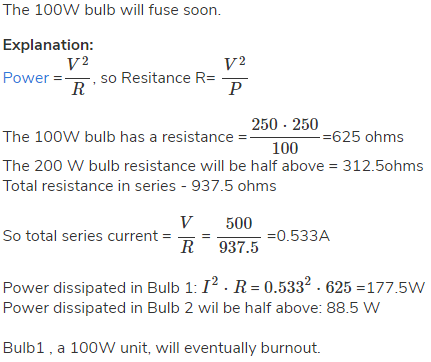Courses

# Two bulbs 100W, 250 V and 200 W, 250 V are connected in series across a 500V line then-a) Both bulbs will be fusedb) 200 W bulb will be fusedc) 100W bulb will be fusedd) No bulb will be fusedCorrect answer is 'C'. Can you explain this answer? Related Test: Test: Electrical Engineering - 2

## Electrical Engineering (EE) Question

Rachika Grewal answered Aug 01, 2018Preety Kumari answered May 23, 2018
A. The 100W bulb has a resistance =250×250/100=625 ohms The 200 W bulb resistance will be half above = 312.5ohms Total resistance in series - 937.5 ohms So total series current = V/R = 500/937.5 =0.533A Power dissipated in Bulb 1: I^2⋅R =( 0.533)^2×625=177.5W Power dissipated in Bulb 2 wil be half above: 88.5 W Bulb1 , a 100W unit, will eventually burnout

By the voltage division rule the voltage across each bulb is 250V. So that in series connection low power capacity bulb glow more and in parallel connection high power capacity bulb glow more.

Shahbaaz Ahmed answered Jul 31, 2018Sourav Sagar answered Apr 19, 2020
C) 100 W bulb will be fused.

A should be the correct answer.

Electrical Department Gp answered Aug 18, 2019
A) should be the correct answer

Vivek Shrivastava answered Feb 10, 2020
Bohot sahi! this is correct explanation!